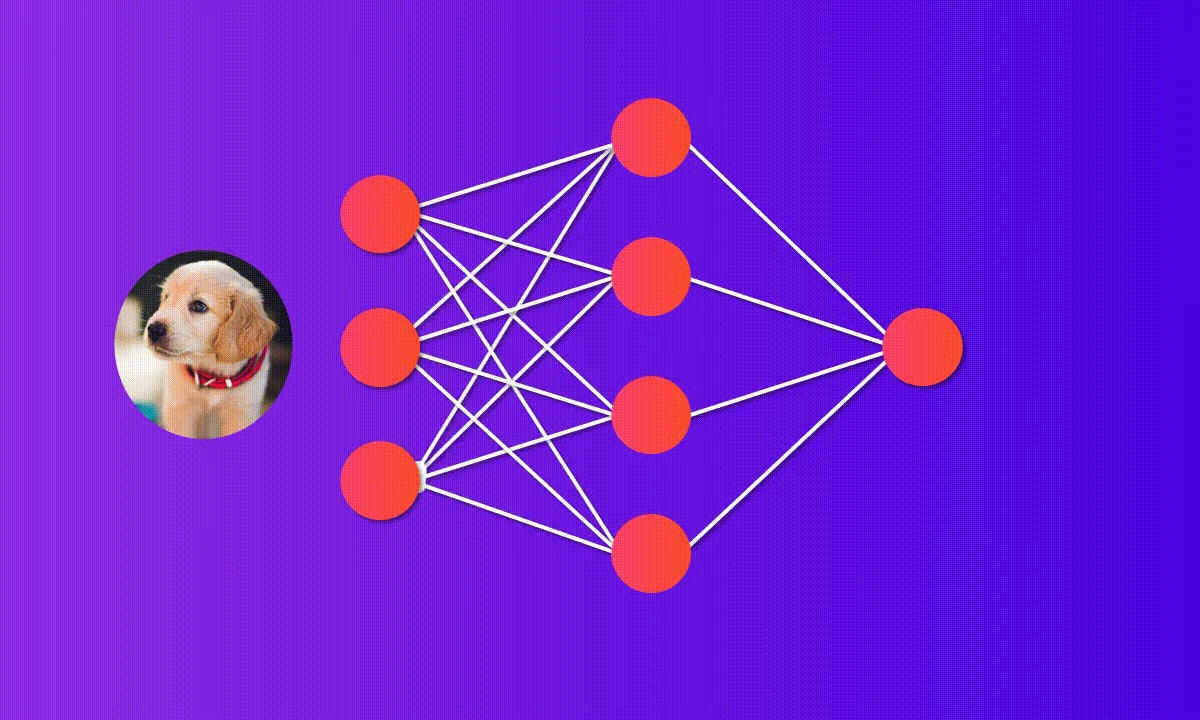# The Deep Learning Story: How this evolved

Source: Deep Learning on Medium# The Deep Learning Story: How this evolved

The universal approximation theorem told us how much the complex relationship between input & output or y=f(x). We can find a neural network such that the output produced (ŷ) would be as close to the true output. Also, backpropagation told us the Deep neural networks are good. Backpropagation is a gradient descent applied with chain rule. Both of these are from 1989–1991 & gradient descent dates back to 1841.

There were some of the challenges in the deep neural network. In backpropagation, we have chain rule of derivative that we apply. So, if we want to update the weight suppose